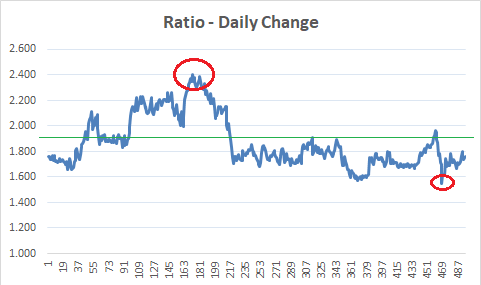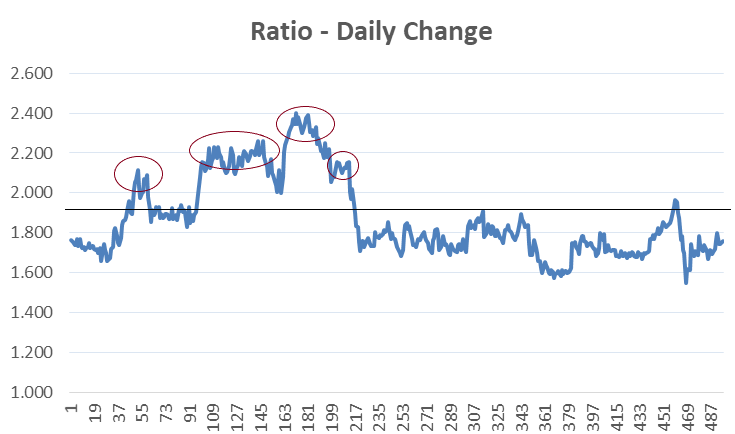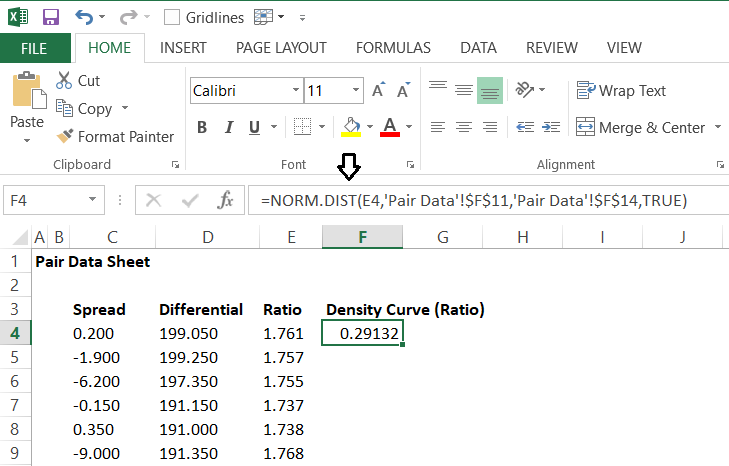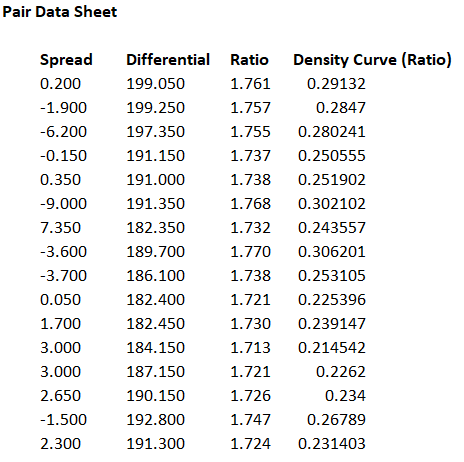# 6. PTM1, C4 – The Density Curve

## 6.1 – A quick recap

I think a quick recap is justified at this stage, this is to ensure we are all on the same page. I’d strongly recommend you read through the recap, to ensure we are on track. I’ll keep this as a pointwise recap to ensure we don’t digress.

• Two companies are comparable if they have similar business background
• Business background includes factors which influence the day to day running of the business
• If two companies have similar business backgrounds, then it is reasonably safe to assume that their share prices move somewhat similarly on a day to day basis
• If the daily stock price of two comparable companies move together (and therefore their daily returns), then they do tend to have a tight correlation
• There are times when a local event can change the course of the movement in the stock price of one of the two companies, creating a pair trading opportunity
• The relationship between the stock prices of the two companies can be estimated by any of the three variables – spread, differential, or ratio
• The variables are expected to be normally distributed, hence we calculate the standard deviation of these variables, along with the basic descriptive statistics such as the mean, median, and mode.
• As a ready reckoner, we also have the standard deviation (SD) table, extending up to the 3rd SD, either sides
• Lastly, do remember we are in the process of discussing two variants of pair trading, starting with Paul Whistler’s technique of Pair Trading. After this, I will discuss a slightly more complicated version of Pair Trading

So this brings us to where we are at this stage. In this chapter, we will go ahead and discuss the density curve and the eventual trigger to pair trade.

## 6.2 – Selecting the variable

We have come to a stage where we need to stick to one of the variables amongst Spread, Differential, and Ratio. Why just and why not all, you may ask?

Well, this is to ensure that we are sticking to a regime and not really getting confused with conflicting signals. The reason I’ve introduced all three variables is to showcase that there are three different possibilities. It is up to you as a trader to choose the variable that you are most comfortable with. For example, I personally prefer the ratio over the differential or spread. This is because the ratio kind of captures the market valuation of the stocks since it considers the latest stock price. Besides the ratio also gives us a quick sense of how much of Stock 1 should be bought or sold with respect to stock 2.

For example, if the price of Stock 1 is 190 and Stock 2 is 80, then the ratio of stock 1 over 2 is –

190/80

= 2.375

This implies for every 1 share of Stock 1, 2.375 shares of Stock 2 has to be transacted.  We will get to the finer details later, but for now, hope you get the drift.

You are of course, free to choose any of the variable – spread, differential, or ratio. However, for the sake of this discussion, I will go ahead with the ratio.

## 6.3 – The trade trigger

As the name suggests, the pair consists of two stock. Until now, we have not defined how to buy or sell a pair, we will do that later in this chapter. For now, assume that you can buy or sell a pair just like the way you can buy or sell a single stock.

As you may have guessed, the decision to buy or sell a pair is dependent on the variable that you track and the variable itself could be the spread, differential, or ratio. For the purpose of this discussion, we are going ahead with the Ratio.

Think about it this way – the stock prices change every day, therefore the ratio of the pair itself changes every day. On most of the days, the daily change in the ratio falls within the expected range. However, there could be days when the daily change goes beyond the expected range. These are the days when a pair trading opportunity arises.

Have a look at the chart below –Casual eyeballing reveals two obvious information –

1. The ratio chart hovers around 1.8 and 2 – probably the ratio’s mean is around this price. I’ve highlighted this with a green line. I’d suggest you check the mean value of the ratio we calculated in the earlier chapters.
2. On most of the days, the ratio hovers above or below the mean value

I want you to pause here and think about this. This is the tipping point in Pair trading, if you can understand everything we have discussed up to now, then the rest is a cakewalk.

The ratio itself is a variable which is derived by dividing stock 1 over stock 2. The ratio changes every day since the stock prices change every day. If you plot the chart of the daily change in the ratio you will notice that the ratio has an average (mean) value and the ratio trades above and below the mean value. Irrespective of where the ratio is today (i.e either above or below the mean) – there is a great chance that ratio will come back to mean over the next few days. Notice, I use the word ‘great chance’, here. This means, that we should be able to quantify the probability of the ratio reverting to mean.

In fact, this phenomenon is referred to as ‘Mean reversion’ or reversion to mean.

I’ve circled (in red) two points in the chart where the ratio has deviated away from the mean. The first circle from the left indicates a point where the ratio has deviated higher than the mean value. The 2nd circle from the left indicates a point where the ratio has deviated below the mean value. In both these cases, eventually, the ratio reverted to mean.

Now, if you look at it in another way – we now seem to have an opinion on the direction in which the ratio is likely will move. For example, the first circle where the ratio has moved above the average indicates that the ratio is likely to retrace back to mean.  Or in other words, you can short the ratio at the high point and buy it back around the mean. Likewise, the second circle points to an opportunity where one can buy the ratio, with an expectation that the ratio will move back to the average value.

Think about the ratio as a stock or futures. Since the directional movement of the ratio is predictable, we can as well place bets on the directional movement of the ratio itself.

I hope you are getting the point here.

The ratio’s value with respect to the mean acts as a key trigger to initiate the trade. If the ratio is –

• Above the mean, the expectation is that the ratio will revert to mean, hence short the ratio
• Below the mean, the expectation is that the ratio will scale back to the mean and hence go long on the ratio

Alright – so far so good. Here are few questions though –

1. The ratio is always above or below the mean value – does this imply there is always a trading opportunity?
2. There are multiple points where the ratio seemed to have bottomed out or peaked, how do we know the exact point at which the trade has to be initiated?

The answers to these questions lie in something called as the ‘Density Curve’. Let’s figure that out.

## 6.3 – The Density Curve

Have a look at the chart below –I’ve highlighted 4 points on the chart, at all these points, the ratio has traded above the mean. Assume, you were looking at this chart around the time the first circle is marked. Now, just because the ratio has shot up above the mean, would you take the trade? In fact, the same question can be asked every time the ratio has traded above (or below) the mean.

I’m sure you’d agree that this would be a great idea. We need to observe the ratio closely and initiate a trade only when the chance of mean reversion is very high. Or in other words, we need to initiate a trade only when we are reasonably certain that the ratio will slide down to the mean value, as quickly as possible.To put the point across – this is pretty much like a tiger waiting in the ambush to hunt down a prey. Just because the prey is in the open, the tiger will not jump and ruin its chances of a kill. It will attack only when it is convinced that the effort will lead to a kill.

So how do we stay in the ambush and wait for our chance for the kill?

Well, we seek refuge in the good old Normal distribution and its properties. I’m hoping you are aware of normal distribution and its properties by now.  Here is a quick recap, I’d suggest you read the complete theory, I’ve discussed this across various chapters in Varsity –

• Within the 1st standard deviation (SD) one can observe 68% of the data
• Within the 2nd standard deviation one can observe 95% of the data
• Within the 3rd standard deviation one can observe 99.7% of the data

So here is what this means with respect to the ratio –

• The ratio, irrespective of where it stands with reference to the mean, has a standard deviation value. For example – it could be just a few points away from the mean and this could translate to say, 0.5 standard deviations from mean
• If the ratio deviates to the 2nd standard deviation, then according to the normal distribution properties, there is only 5% chance of it going higher or in a very loose sense, it poses a 95% chance of reverting to mean.
• Likewise, if the ratio deviates to the 3rd standard deviation, then it only has a 0.3% chance of drifting higher or in a very loose sense, it poses a 99.7% chance of reverting to mean

So at every SD, we can estimate the likelihood of the ratio reverting to mean. This means we can filter out opportunities and initiate a trade only at points where the likelihood of success is high.

This further leads to an interesting take – the key trigger to initiate a trade is not just based on where the ratio is, but also depended on its standard deviation. Given this, it makes sense to directly track the daily standard deviation of the ratio as opposed to the ratio itself.

This can be achieved by tracking the ‘Density Curve’ of the ratio. The density curve is a non-negative value which lies anywhere between 0 and 1. I’d suggest you watch this video on Khan Academy to learn more about Density Curve.

Calculating the density curve on excel is quite straightforward. Here is how you can do this, have a look at the image below –You can use the inbuilt excel function called Norm.dist for this. The function requires 4 inputs –

• X – this is the daily ratio value
• Mean – this is the mean or average value of the ratio
• Standard Deviation – this is the standard deviation of the ratio
• Cumulative – You have to select true or false, select the default value as true.

I’ve calculated the density curve value for all variables, here is how the table looks –I guess we could break this chapter at this point. In the next chapter, we will look into details on how we can use the density curve to trigger long and short pair trade.

### Key takeaways from this chapter

• Ratio as a variable is more versatile as it captures the valuation elements of the stock
• The ratio tends to trade above or below its mean value
• The idea is the ratio, when it deviates away from the mean, will also tend to revert to mean
• At every point at which the ratio deviates, we can measure the probability of its reversion to mean
• The above point can be measured by normal distribution
• The density curve is a non-negative value which varies between 0 and 1. This can be easily calculated on MS Excel by using an in build function.

1.Pradeep says:

Sir that was a very dense chapter, but your explanation made all the difference. Thank you. I have a question. Going by what I understand, I’d guess the system wouldn’t generate more than 2 or 3 trades per month, assuming we have tracked 3-4 pairs. The opportunity wouldn’t be frequent since other traders would be looking for it too. Am I right in saying that?

•Karthik Rangappa says:

Yes, the pair trading opportunities do not come by often. Also, it really depends on how the kind of technique you are adopting to pair trade. More on this later.

•Kuldeep says:

Sir, can you suggest any indicator which also works as good as TRIN and PCR in zerodha. Thank You

•Karthik Rangappa says:

Each indicator has its own set of pros and cons, hence very difficult to isolate one indicator and call it the best.

2.Deepu says:

Excellent write-up!!!
Million thanks for writing this complex subject in the lucid manner.

Thanks once again.

•Deepu says:

•Karthik Rangappa says:

Got it 🙂

•Karthik Rangappa says:

Will try and put this up soon 🙂

•Rushabh Doshi says:

programming skills in which software would help in trading?

•Karthik Rangappa says:

I’ve heard Python is a great language for traders.

3.Akash Patel says:

hello sirji,
good enlighten about density curve…i heard about it first time…though doing pair trading since last one and half year when ratio reach to +/- 2 SD…let’s see what will come next!!
thanks bro…keep it up.

•Karthik Rangappa says:

Glad you liked it 🙂 Will try and put up the next chapter as soon as possible.

4.D.Omprakash says:

I have downloaded DLF 5 years data and placed in price column in excel which you have provided but nothing seems to changed means nothing displays.Please help to sort out this issue.

•Karthik Rangappa says:

Like I said, kindly check if the cells are properly linked.

5.Pramod doke says:

Sir..
Particularly using MS-Excel.

•Karthik Rangappa says:

Planning to write a module on Financial Modelling using MS-Excel.

•Pramod doke says:

Thanks sir..
That will be indeed great help…

•Karthik Rangappa says:

Cheers!